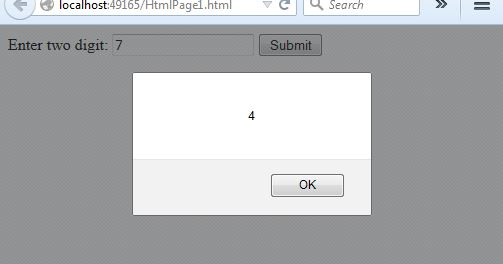#### JavaScript > Assignment Operators

##### How to divide, get remainder and assign value easily in JavaScript?

To divide, get remainder and assign value easily, we can follow this approach.

```<script>
function Divisionremainder() {
var a = document.getElementById("txtA").value;
var result = 25;
result %= parseInt(a);
}
</script>

Enter two digit:
<input type="text" id="txtA" name="txtA" />
<input type="button" name="btnDivisionremainder" value="Submit"
onclick="Divisionremainder()" />
```

In the above code snippet, we have one textbox. On click of button, we are calling the `Divisionremainder`function that divide, get remainder, assign the number and show result in the alert. Like in previous post, here also the text box value has been used to parse the text box data into integer.

OUTPUTViews: 2722 | Post Order: 23